### Home > CALC > Chapter 1 > Lesson 1.5.1 > Problem1-202

1-202.
1. Find the domain of the following functions: Homework Help ✎

1. f(x) = sec x

2. g(x) = log(x2 + l)

3.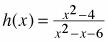4.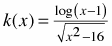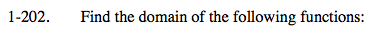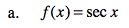When is the cos(x) = to 0?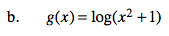Logarithms have restrictive domains: x cannot be less than or equal to 0. What value(s) of x will make x² + 1 ≤ 0?

D = (−∞, ∞)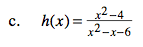x² - x - 6 ≠ 0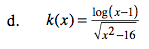Both the numerator and the denominator have restricted domains. To find the domain of k(x), all restrictions apply... combine the domains together.